Automation, Control and Intelligent Systems
Volume 4, Issue 6, December 2016, Pages: 95-100

Control of Flexible Joint Robot Using Integral Sliding Mode and Backstepping

Sungha Kwon, Abner Asignacion, Seungkyu Park

School of Electrical Electronics & Contol, Changwon National University, Changwon, Gyeongnam, South Korea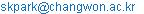(SeungKyu Park)

Sungha Kwon, Abner Asignacion, Seungkyu Park. Control of Flexible Joint Robot Using Integral Sliding Mode and Backstepping. Automation, Control and Intelligent Systems. Vol. 4, No. 6, 2016, pp. 95-100. doi: 10.11648/j.acis.20160406.13

Received: December 6, 2016; Accepted: December 20, 2016; Published: January 16, 2017

Abstract: The control of flexible joint robot is getting more attentions because its applications are more frequently used for robot systems in these days. This paper proposes a robust impedance controller for flexible joint robots by using integral sliding mode control and backstepping control. The sliding mode control decouple disturbances completely but requires matching condition of disturbances. The dynamic model of FJR is divided into motor side and link side and the disturbance of the link side does not satisfy matching condition and cannot be decoupled directly by the actual input in the motor side. To overcome this difficulty, backstepping control technique is used with sliding mode control. The mismatched disturbance in the link side is changed into matched one in the respect to virtual control input which is the state controlled by actual input in the motor side. Integral sliding mode control is used to preserve the impedance control performance and the improved robustness at the same time.

Keywords: Flexible Joint Robot, Backstepping Control, Integral Sliding Mode, Mismatched Disturbance, Robust Impedance Control

Contents

1. Introduction

Nowadays, control of flexible joint robots (FJR) are getting increasing interests [1-5]. Flexibility in robot joints comes from flexible mechnism like series elastic actuator, belt-pully transmission and harmonic drives. Because of the variety of robotic field, the control of FJR is becoming very important topics.

Main topic of this paper is to propose a robust controller which can decouple disturbances in FJR. There are representative robust control methods such ascontrol [6-7], disturbance observer (DOB) [8-9], adaptive control [10-11] and sliding mode control (SMC) [12-15]. Among them, sliding mode control decouples disturbances almost completely through the sliding mode. So it is desirable to use SMC for the control of FJRs which have disturbances. However, matching condition is required for the application of SMC but FJR does not satisfy this condition.

To overcome this difficulty, backstepping control technique is used with SMC. The FJR model is considered as two models: link side and motor side. In the backstepping control of FJR, the motor side angle position is considered as a virtual controller for the link side system and actual control input in the motor side is designed to achieve the desired motor side angle position. There are some existing results which use backstepping control for FJR but deos not consider SMC and backstepping together [16-19].

Besides robustness, the control performance must be considered in the control of FJR. To have desirable impedance is one of the frequently consdered control performance in robotic field.

Integral sliding mode control makes it possible to achieve this control performance and robustness together. The dynamic charcteristic of desired impedance can be involved in a integral sliding surface [20-24]. The reaching phase problem is naturally disapeared by choosing the initial virtual state in the integral sliding surface. The link side impedance is chosen as the overall impedance of FJR and the motor side impedance is dertermined high enough.

The impedance controllers are designed first and they are capsulized by integral sliding surfaces. An ISMC is designed for the link side first and then for the motor side based on Lyapunov stability.

Ths paper organized as follows. chaper 2 formulates the problem to be solved. The basics of ISMC and backstepping control are discussed in chapter 3 and their composition and apllication to FJR is considered in chapter 4. Simulation results are shown in chapter 5 and conclusions are given in Chaper 6.

2. Problem Formulation

Consider the following dynamics of a flexible joint robot: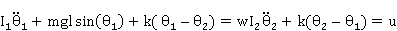(1)

where u is the torque input, w is the disturbance, I1 is the link inertia, I2 is the motor inertia, m is the mass, g is the gravitational acceleration, l is the link length, k is the stiffness constant and θ1 and θ2 are angular positions of link side and motor side respectively.

Set x11, x2=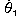, x32 and x4=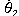, then (1) is expressed as follows: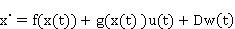(2)

where,.

It is desired to improve the robustness of the system against the disturbance by using the SMC, but the matching condition is not satisfied as shown in the difference of g and D in (2). To overcome this difficulty, the backstepping control is used with SMC in this paper.

3. ISMC and Backstepping

The ISMC has the robustness of the original sliding mode control and the performance of a nominal system without the effect of existing uncertainties. The ISMC input consists of two parts.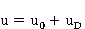(3)

where the term u0 is the nominal controller generated by a high level controller (which can be designed according to any suitable design method), while uD is a discontinuous control action designed to reject the matched perturbation terms, forcing the states stay on a suitably designed sliding manifold s=0.

The integral sliding manifold is defined as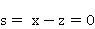(4)

where z is chosen to have the following dynamic.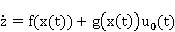(5)

In the implementation of the ISMC, the system can be on the sliding surface from the initial time, i.e., by choosing z(0) satisfying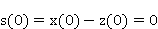(6)

Under the ISMC, the system behaves as like nominal system which does not have any disturbance or uncertainty. On the sliding surface the following is satisfied.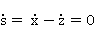(7)

then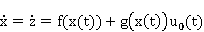(8)

This shows that the system has nominal characteristics on the sliding surface. To derive the ISMC input through the Lyapunov stability, let the Lyapunov candidate function be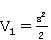(9)

The sliding function s converges to zero if the following condition is satisfied.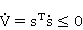(10)

The followings show how to derive an ISMC input to satisfy the above condition.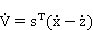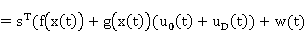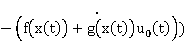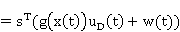Considering the equation above, complying with the matching condition, the discontinuous input that makes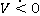is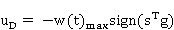(11)

where g is a common matrix of the input and the perturbations.

The ISMCs reject the matched perturbations only. But as shown in (2), the link side disturbance does not comply with the matching condition. To apply the ISMC to the FJR, backstepping control must be used with ISMC.

For backstepping design, the overall system must be divided into cascaded parts and expressed in strict-feedback form as(12)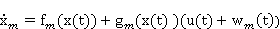where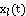is link side state and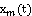is motor side state,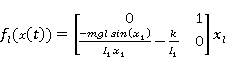,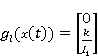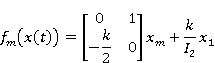As part of the backstepping procedure, a state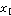is virtually controlled by the state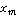. Then, the actual statemust follow that stabilizing virtual stateand this stabilizing virtual control can be denoted as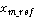. This tracking control objective is described as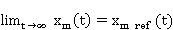(13)

The actual control input used to achieve the above control objective. The detail explanations for the input derivation of the FJR will be shown in chapter 4.

4. Control of FJR Using ISMC and Bacstepping

Backstepping control divides the FJR dynamics into two cascaded local systems. Then, the actual torque input controls the state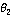and then recursively the statecontrols the state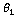which is the output of the FJR.

To achieve impedance control, the following nonlinear state decoupling and state feedback are applied.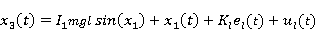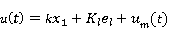(14)

where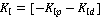are impedance gains for link side and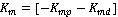are impedance gains for motor side.

Then error equations are as follows.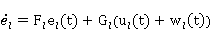(15)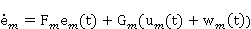where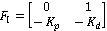,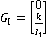,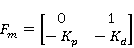,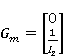.

For the link side system, the proposed integral sliding surface is defined as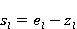(16)

where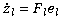And for the motor side, the sliding surface is defined as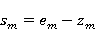(17)

where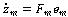In the first step, the desired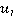is derived based on the ISMC. The first Lyapunov candidate function is chosen as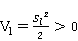(18)

Its time derivative is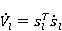By substituting (15) and (16) into the above equation,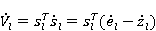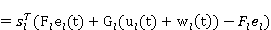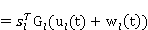To ensure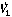to be less than zero, the discontinuous control input is determined as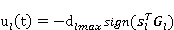(19)

As a result,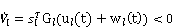(20)

Actually the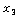derived in this step is the reference input of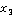and it can be denoted as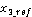. In (14),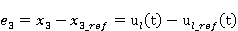(21)

The second Lyapunov candidate function is chosen as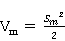(22)

The total Lyapunov candidate function can be defined as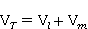(23)

Its time derivative is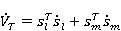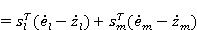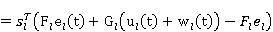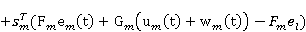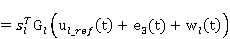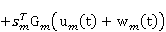To ensureis less than zero, the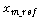and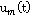are determined as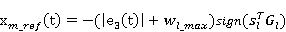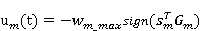(24)

5. Simulation Results

This chapter presents the simulation results. The parameter values used in simulation are shown in Table 1.

Table 1. Flexible Joint Robot parameter.

 Quantity Value k 100Nm/rad m 1kg I1 1kgm2 I2 1kgm2 l 1m g 9.8m/s2

Impedance gains are given as follows.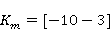The following disturbances are assumed.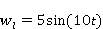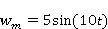And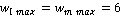.

Figure 1 shows the outputs of the FJR system for the backstepping impedance control for the case of nominal system and the case of existing disturbance.Figure 1. Output Response of FJR with Impedance Controller.

Figure 1. Shows that backstepping impedance control performance is affected by disturbances.

Figure 2. Shows the output of the FJR controlled by proposed controller in the case of mismatched disturbance.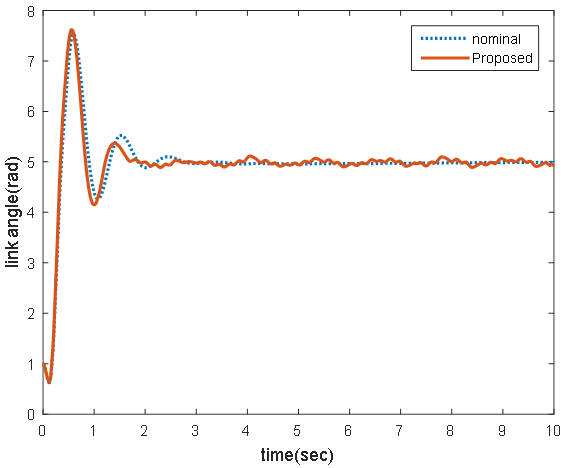Figure 2. Output Response of FJR with proposed Controller.

The Figure 2 shows that the FJR output controlled by the proposed controller is not affected by mismatched disturbance.

The Figure 3 shows the backstepping impedance control input.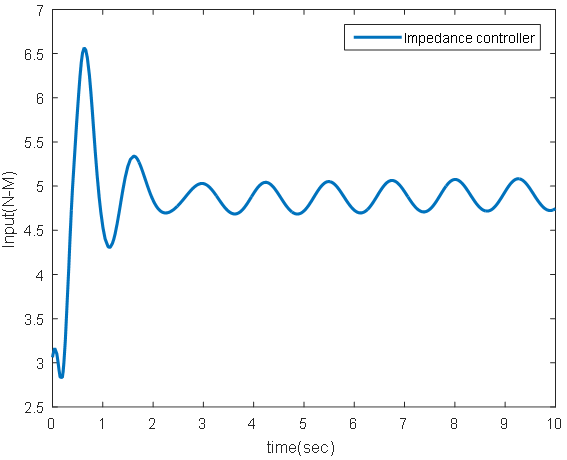Figure 3. Impedance Control Input.

The Figure 4 shows the proposed control input signal which shows chattering caused by SMC.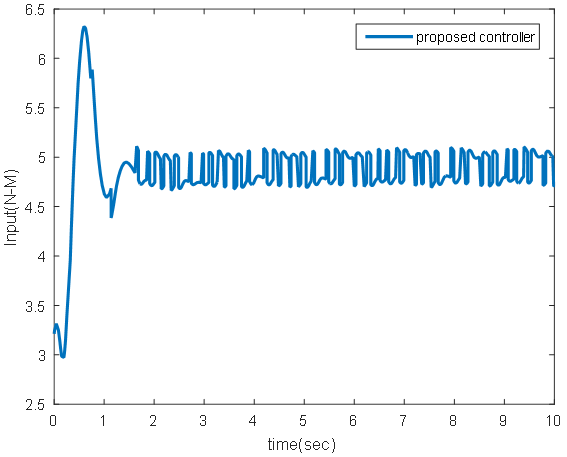Figure 4. Proposed Control Input.

The robustness of the proposed controller for bounded mismatched disturbance is demonstrated for a bounded mismatched disturbance.

6. Conclusion

The new robust impedance controller with ISMC and backstepping control scheme is proposed for the flexible joint robot with mismatched perturbations. The backstepping is introduced to solve mismatched perturbations in the link side and the proposed controller has the performance of nominal impedance control in the case of mismatched disturbances in the link side.

Acknowledgements

This paper was supported by Changwon National University Research Fund in 2015~2016.

References

1. G. Pratt and M. Williamson, "Series elastic actuators," in 1995 IEEE/RSJ International Conference on Intelligent Robots and Systems (IROS), vol. 1, pp. 399406, Aug. 1995.
2. A. Albu-Schaffer, O. Eiberger, M. Grebenstein, S. Haddadin, C. Ott, T. Wimbock, S. Wolf, and G. Hirzinger, "Soft robotics," Robotics & Automation Magazine, IEEE, vol. 15, no. 3, pp. 2030, Sep. 2008.
3. S. Ozgoli, H. D. Taghirad, "A Survey on the Control of Flexible Joint Robots," Asian Journal of Control, Vol. 8, No. 4, pp. 332-344, Jul. 2006.
4. S. Ulrich, J. Sasiadek, "Extended Kalman Filtering for Flexible Joint Space Robot Control," 2011 American Control Conference, pp. 1021-1016, June 29 - July 01, 2011.
5. Kostas Nanos, G. Papadopoulos, "On the dynamics and control of flexible joint space manipulators," Control Engineering Practice Control Engineering Practice, vol. 45, pp. 230–243, 2015.
6. F. Wang, X. Liu, "Fuzzy H-inf Control of Flexible Joint Robot," Information Computing and Applications Lecture Notes in Computer Science, vol. 7030, pp. 424-431, Oct. 2011.
7. L. Bakule, B. Rehak, M. Papík, "Decentralized H-infinity control of complex systems with delayed feedback," Automatica vol. 67, pp. 127-131 May 2016.
8. M. J. Kim, W. K. Chung, "Robust Control of Flexible Joint Robots Based On Motor-side Dynamics Reshaping using Disturbance Observer (DOB)," IEEE/RSJ International Conference on Intelligent Robots and Systems, pp. 2381-2388 2014.
9. Z. Yang, B. Meng, H. Sun, "A new kind of nonlinear disturbance observer for nonlinear systems with applications to cruise control of air-breathing hypersonic vehicles," International Journal of Control, pp. 1-16, Sep 2016.
10. R. Lozano, B. Brogliato, "Adaptive Control of Robot Manipulators with Flexible Joints," IEEE Transactions on Automatic Control, vol. 37, no. 2, pp. 174-181, Feb. 1992.
11. F. Ghorbel, J. Hung, M. Spong, "Adaptive Control of Flexible-Joint Manipulators," IEEE International Conference on Robotics and Automation, pp. 1188-1193, 1989.
12. A. Hace, K. Jezernik, and A. Sabanovic, "SMC with disturbance observer for a linear belt drive," IEEE Transactions on Industrial Electronics, vol. 54, no. 6, pp. 3402-3412, Dec. 2007.
13. V. Utkin, J. Shi, "Integral sliding mode in systems operating under uncertainty conditions," Proc. 35th IEEE Conference on Decision Control, Kobe, Japan, pp. 4591-4596, Dec. 1996.
14. L. E. Jiménezm, A. Loukianov, E. B. Corrochano, "Integral Nested Sliding Mode Control for Robotic Manipulators," 17th World Congress The International Federation of Automatic Control, Seoul, Korea, July 6-11, pp. 9899-9904, 2008.
15. M. Rubagotti, F. Castaños, A. Ferrara and L. Fridman, "Integral Sliding Mode Control for Nonlinear Systems With Matched and Unmatched Perturbations," IEEE Transactions on Automatic Control, vol. 56, no. 11, pp. 2699-2704, Nov. 2011.
16. Y. Lin, C. Cheng, "Design of adaptive backstepping tracking controllers for a class of mismatched perturbed chaotic synchronization systems," SICE Annual Conference, pp. 2549-2544, 2010.
17. J. H. Oh and J. S. Lee, "Backstepping control design of flexible joint manipulator using only position measurement," Proc. IEEE Conference on Decision Control, vol. 1, pp. 931-936, Dec. 1998.
18. Y. Chang, "A Regressor Free Adaptive Backstepping Design of Flexible Joint Robot Based on Function Approximation Technique," First International Conference on Robot, Vision and Signal Processing, pp. 3435-3440, Apr. 1997.
19. J. Oh, J. Lee, "Control of Flexible Joint Robot System by Backstepping Design Approach," International Conference on Robotics and Automation, pp.131-136, 1997.
20. P. Tomei, "A Simple PD Controller for Robots with Elastic Joints," IEEE Transactions on Automatic Control, vol. 36, no. 10, pp. 1208-1213, Oct. 1991.
21. A. De Luca, E. Guglielmelli, and P. Dario, "Compliance control for an anthropomorphic robot with elastic joints: Theory and experiments," Journal of dynamic systems, measurement, and control, vol. 127, no. 3, pp. 321-328, Jul. 2005.
22. A. De Luca, B. Siciliano, and L. Zollo, "PD control with on-line gravity compensation for robots with elastic joints: Theory and experiments," Automatica, vol. 41, no. 10, pp. 18091819, Oct. 2005.
23. M. J. Kim and W. K. Chung, "Design of nonlinear optimal impedance controllers," in IEEE/RSJ International Conference on Intelligent Robots and Systems (IROS), pp. 1972-1978, 2013.
24. J. H. Kim, S. Andrew Gadsden and Stephen A. Wilkerson, "Adaptive integral sliding mode controller for longitudinal rotation control of a tilt-rotor aircraft," 24th Mediterranean Conference on Control and Automation (MED), pp. 820-825, Jun. 2016.

 Contents 1. 2. 3. 4. 5. 6.
Article ToolsAbstractPDF(267K)